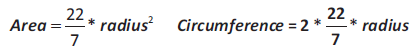# Java program to input the radius of a circle and find its area and circumference using math power function

Write a program to input the radius of a circle and find its area and circumference.`Solution`

``````import java.util.*;
class AreaCircle
{
public static void main(String arr[])
{
double c,a,r;
Scanner sc=new Scanner(System.in);
System.out.println(“Enter the radius of the circle:”);
r=sc.nextDouble();
a=22/7.0 * Math.pow(r,2);
c=2*22/7.0*r;
System.out.println(“Area=”+a);
System.out.println(“Circumference=”+c);
}
}``````
`Output`